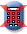Massachusetts Comprehensive Assessment System

Question 4: Constructed-ResponseReporting Category: Measurement and Data
Standard: Mathematics.5.MD.C.04 - Measure volumes by counting unit cubes, using cubic cm, cubic in., cubic ft., and non-standard units.
Item Description: Determine the volume of right rectangular prisms by counting unit cubes and using volume to solve problems.

View item in MCAS Digital Item Library

## Scoring Guide and Sample Student Work

Select a score point in the table below to view the sample student response.

ScoreDescription
4 The student response demonstrates an exemplary understanding of the Measurement and Data concepts involved in measuring volumes by counting unit cubes, using cubic cm, cubic in., cubic ft., and improvised units. The student correctly finds the volume of figures in 1-inch cubic units and compares the volumes.
4
3 The student response demonstrates a good understanding of the Measurement and Data concepts involved in measuring volumes by counting unit cubes, using cubic cm, cubic in., cubic ft., and improvised units. Although there is significant evidence that the student was able to recognize and apply the concepts involved, some aspect of the response is flawed. As a result, the response merits 3 points.
2 The student response demonstrates a fair understanding of the Measurement and Data concepts involved in measuring volumes by counting unit cubes, using cubic cm, cubic in., cubic ft., and improvised units. While some aspects of the task are completed correctly, others are not. The mixed evidence provided by the student merits 2 points.
1 The student response demonstrates a minimal understanding of the Measurement and Data concepts involved in measuring volumes by counting unit cubes, using cubic cm, cubic in., cubic ft., and improvised units.
0 The student response contains insufficient evidence of an understanding of the Measurement and Data concepts involved in measuring volumes by counting unit cubes, using cubic cm, cubic in., cubic ft., and improvised units to merit any points.
Note: There are 2 sample student responses for Score Point 4.

Question 4: Measurement and Data
Question 13: Number and Operations in Base Ten

Last Updated: May 5, 2020